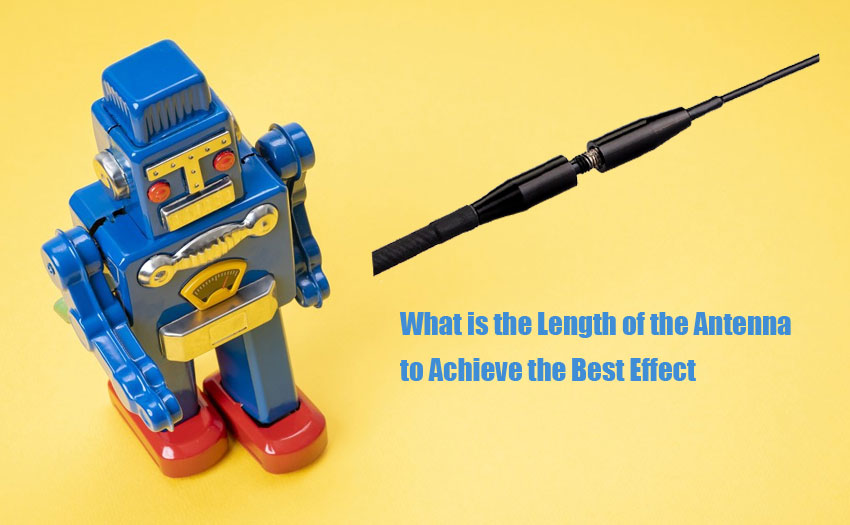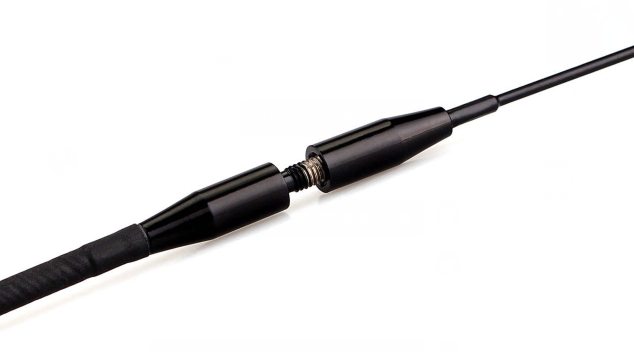# What is the Length of the Antenna to Achieve the Best EffectWhen many "hams" install and make their own antennas, they feel that the longer the antenna, the more obvious the effect of transmitting or receiving signals. In fact, the longer the antenna is, the better. Only in the most suitable range can the antenna function to the greatest extent. We know that there are some parameters that can affect the transmission or reception characteristics of the antenna, such as direction, weather, surrounding environment, quality, installation method, life, noise temperature, etc. The length of the antenna is also a very important factor.

So, what is the length of the antenna to achieve the best effect?
Theory and practice have proved that when the length of the antenna is 1/4 of the wavelength of the radio signal, the transmission and reception conversion efficiency of the antenna is the highest. Therefore, the length of the antenna will depend on the frequency or wavelength of the transmitted and received signals. As long as you know the center frequency of the corresponding transmission and reception, you can use the following formula to calculate the wavelength of the corresponding radio signal, and then divide the calculated wavelength by 4 to obtain the optimal antenna length for the pair.

The conversion formula for frequency and wavelength is:

Wavelength = 300,000 km/frequency

= 300000000 meters/frequency (the resulting unit is meters)

Example: Find the antenna length of an amateur radio station. It is known that the signal frequency used by the amateur radio station is around 432MHz, and its wavelength is:

Wavelength=300000km/435MHz

=300000000/435000000

=300/435

=0.69m

The corresponding optimal antenna length should be 0.69/4 equal to 0.1725 meters

When the frequency is 439MHz, you can simplify the calculation formula as

Wavelength=300/439

=0.683m

The optimal antenna length is 0.683 m/4, which is equal to 0.17 m.